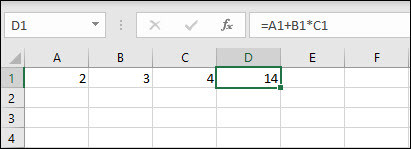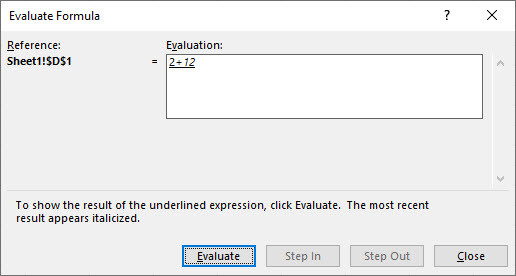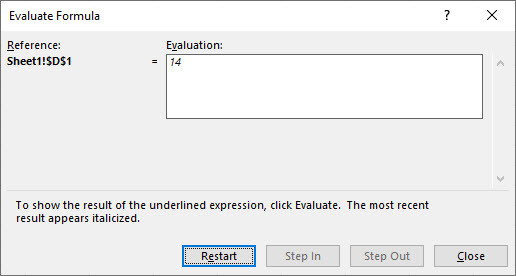Search

# How to break down a formula in Excel

Updated: Sep 10, 2020

Do you sometimes struggle to understand how a formula works in Excel? The good news is with the Evaluate Formula tool, you can break it down into simple steps so you can see how each part is calculated and the order in which it's done. This is really useful when you're trying to understand a complex formula that's been written by someone else.

Let's see how it works with what on the surface appears to be a simple formula:

What we want to do is add A1 and B1 together and multiply the result by C1.

If we use the numbers from the cells and work it out on a calculator, we'll get 20 for the formula result. However, if we create the same calculation in Excel, we get 14! Why is that?Let's use the Evaluate Formula tool to find out:

First, select the cell that contains the formula you want to evaluate, then click the Formulas tab on the ribbon and select Evaluate Formula.This will open the Evaluate Formula window and you'll see your formula appear in the Evaluation box. The underline will show you which part of the formula will be evaluated next (cell A1 in this example).Click Evaluate to start the process. Excel will now display the value that is stored in Cell A1, and then move on to the next part of the formula. Click Evaluate to move to the next step.Excel displays the value stored in cell B1 and moves on to the next part of the formula. Click Evaluate again.Excel displays the value stored in cell C1 and moves to the next part of the formula.

Click Evalute again.So now Excel has all the relevant numbers, it can solve the formula. Surprisingly, Excel does not perform the addition first - it does the multiplication (3x4)! That's because there is a hierarchy when it comes to mixing operators, and multiplication comes higher up the list than addition.

Click Evaluate again. So Excel works out 3x4 and it's now ready to perform the final part of the calculation.Click Evaluate one final time to view the formula result.

So that's why we got 14 and not 20!If you want to start the process again you can click Restart, otherwise click Close.

So there it is - an easy way to understand how your formulas are calculated.

Why not try it out on one of your own formulas?